﻿ Data Fitting BasicsHome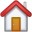Return to the GraFit home page

Product Info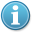Get information about the GraFit program

Technical SupportGet technical support information about the GraFit program

Purchase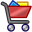Get information about purchasing GraFit software licensesDownload the GraFit program and program updates

Search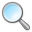Search the GraFit web site

Site map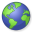View the structure of the GraFit web site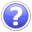Get information about Erithacus SoftwareHome

Technical

Sales

Web Site

Home >  Product information > Data Fitting Basics

### Data Fitting Basics

This extract from the GraFit user manual describes some of the basic principles behind data fitting.

### Regression Analysis

Regression analysis finds the "best fit" line or curve through a series of data. This is considered to be the one that minimizes the sum of the squares deviations of the experimental data points from the theoretical curve. GraFit uses regression to allow the analysis of experimental data.

#### The Errors are in the y Data

It is the convention to assume that the x data values represent the independent variable, and the y data values represent the dependent variable, i.e. the x data are what is varied in the experiment (for example time, concentration, etc.), and the y data are the experimentally observed values.The vertical distance (the red lines in the above diagram) between the data point and the curve or line is that which is minimized by normal regression analysis, i.e., it is assumed that all errors are present in the y data. An important aspect of experimental design is to ensure that the x data are as accurate as possible so that this assumption is valid.

#### The Data Values Have A Suitable Numerical Range

A consequence of computer calculation is that rounding errors can occur, particularly if very large or very small numbers are used. This is because computers only store decimal numbers with a limited numerical precision. Although this should not affect most calculations, it is advisable to scale any set of very large or very small values prior to performing calculations. For example, if a series of time values were entered as 0 to 10-15 seconds, the rounding errors could be significant. Entering the data as 0 to 1 femtoseconds may be more appropriate.

### Types of Regression Analysis

Regression acts to minimize the sum of the squares deviations of the experimental values from values calculated using some theoretical equation. However, the mechanics of the calculations are different for linear and non-linear equations.

#### Linear Regression

Linear regression is a technique that should be familiar to most scientists. It is the mathematical equivalent of using a ruler to draw the "best" line through a series of data points to obtain values for the slope and intercept of this line. The calculations needed to perform linear regression are relatively simple, and are even found on many scientific calculators.

#### Polynomial Regression

Polynomial regression is an extension of linear regression, where the equation is

y = a + bx + cx2 +dx3 + ex4 + ...

#### Non-linear Regression

Non-linear regression allows data to be fitted in the more general case to any equation where the y data value can be described as a function of the x data and a series of parameters, i.e.

y = f(x, p1, p2, p3, ...)

As a result, it is applicable to many more situations than linear regression, and is more generally useful for analyzing experimental data. The only drawback to non-linear regression is that the calculations are too complex perform using a calculator, and require a computer program such as GraFit.

### Additional Considerations for Non-linear Regression

The more complex nature of the non-linear regression calculations do require some further considerations compared with linear regression.

#### Equation to Use

Non-linear regression can employ a vast range of different equations. It is therefore necessary to select an equation that is appropriate to the particular experimental situation before fitting the data.

#### Initial Estimates

The mathematical method used to perform non-linear regression acts to take starting estimates for the parameters in the equation, and optimize them during the calculations. GraFit provides a mechanism for providing these initial estimates automatically for some equations, but in principle it is necessary to give the calculations some rough estimates prior to data fitting.

#### Convergence Criteria

Unlike linear regression, non-linear regression does not provide an exact solution, but, by an iterative process, calculates successively better parameter values until a suitable tolerance is reached. The criteria for defining this tolerance are determined by the program, and can be specified when using GraFit.

### Weighting Scheme

Both linear and non-linear regression analyses incorporate assumptions about the accuracy of the data points. Normally, they assume that all data points are equally accurate, and so carry equal weighting in the analysis. It is also possible to use other weighting schemes that give different weighting to one or more data values.

### Linear Regression of Transformed Data

A familiar method of analyzing non-linear data graphically is to rearrange the data to a linear form, find the "best line" through the points, and so derive the parameter values. This is illustrated in the following example. Enzyme kinetic data are described by an equation of the form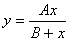which is hyperbolic. This can be linearized by plotting 1/y versus 1/x, as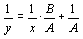The ease of linear regression analysis makes it tempting to use in order to fit data that have been rearranged in this way. However, the results that are obtained are not statistically optimal. This is because the regression calculations make assumptions about the error distribution that are not usually valid after transformation. The distortion of the experimental errors by the above transformation is easily demonstrated in the following graphs.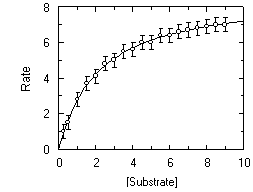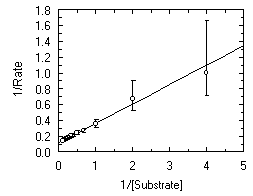Enzyme kinetics plot. The error is present only in the Rate (y axis) measurement. Errors are simple, i.e. all data points have the same error. Double reciprocal (Lineweaver Burk) plot of the data above. Note the large distortion of the error bars resulting from this plot.

In this case, the error involved in the high data points of the transformed plot is much greater than in the low data points - this is simply a consequence of the reciprocal transformation. Linear regression (unless suitable compensation is made for the distorted errors) is therefore not an appropriate way to analyze the data, and for best results the original data should be fitted to the appropriate non-linear equation.

### Information Obtained From Data Fitting

Data fitting provides the following information regarding the experimental data that are analyzed.

1. The values of the parameters in the equation used that best describe the data. These parameters may include (for example) rate constants, inhibition constants etc. whose values need to be determined.

2. The accuracy of these calculated parameters.

From this information it is possible to judge how well the data fit the experimental model (equation) that was used. In most cases, however, it is the numerical values of the parameters that are important, and whose values are required from the analysis.

 Terms of Use  •  Privacy Policy  •  Contact Us Copyright © Erithacus Software Limited, 2010